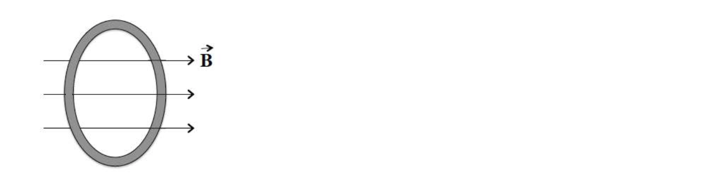# Problem: A circular loop of wire of radius L is in a uniform magnetic field, with the plane of the loop perpendicular to the direction of the field. The magnetic field varies with time according to B(t) = a + bt, where a and b are constants. a) Calculate the magnetic flux through the loop at t = 0. b) Calculate the emf induced in the loop. c) If the resistnace of the loop is R, what is the induced current? d) At what rate is energy being delivered to the resistance of the loop?

###### Problem Details

A circular loop of wire of radius L is in a uniform magnetic field, with the plane of the loop perpendicular to the direction of the field. The magnetic field varies with time according to

B(t) = a + bt, where a and b are constants.

a) Calculate the magnetic flux through the loop at t = 0.

b) Calculate the emf induced in the loop.

c) If the resistnace of the loop is R, what is the induced current?

d) At what rate is energy being delivered to the resistance of the loop?